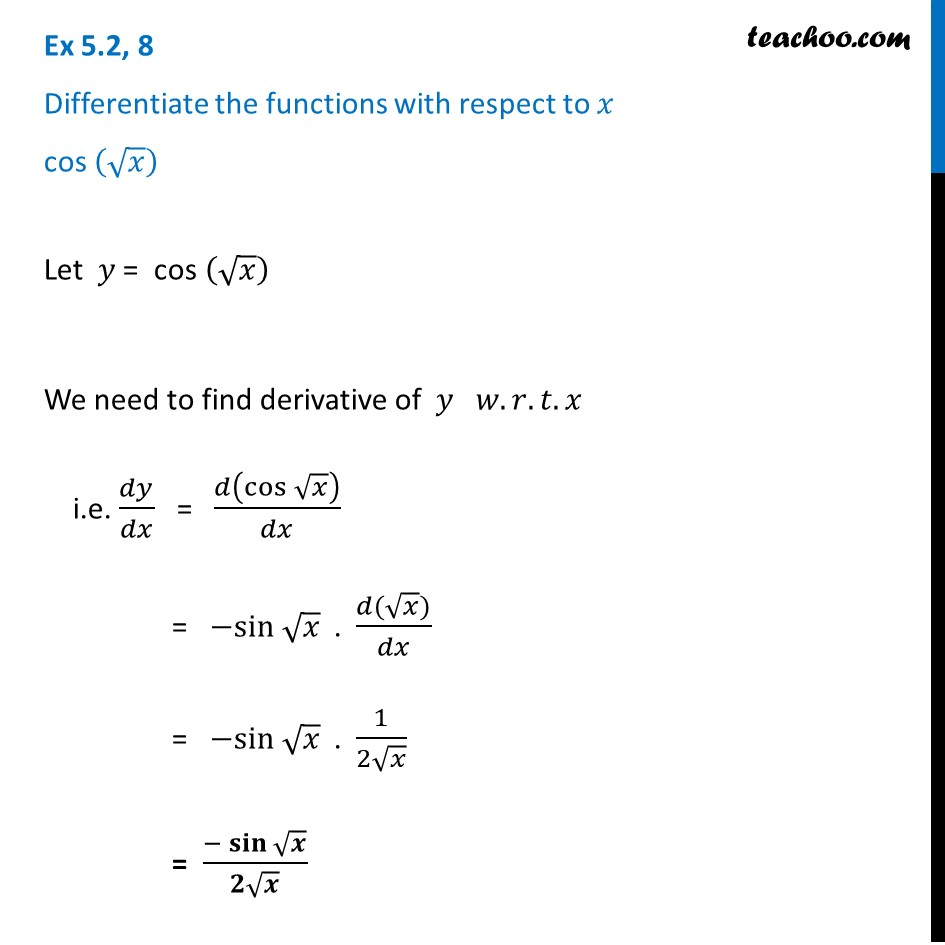Ex 5.2

Chapter 5 Class 12 Continuity and Differentiability
Serial order wiseLearn in your speed, with individual attention - Teachoo Maths 1-on-1 Class

### Transcript

Ex 5.2, 8 Differentiate the functions with respect to 𝑥 cos (√𝑥) Let 𝑦 = " cos " (√𝑥) We need to find derivative of 𝑦 𝑤.𝑟.𝑡.𝑥 i.e. 𝑑𝑦/𝑑𝑥 = 𝑑(cos⁡√𝑥 )/𝑑𝑥 = −sin √𝑥 . (𝑑(√𝑥))/𝑑𝑥 = −sin √𝑥 . 1/(2√𝑥) = (−𝐬𝐢𝐧⁡√𝒙)/(𝟐√𝒙)